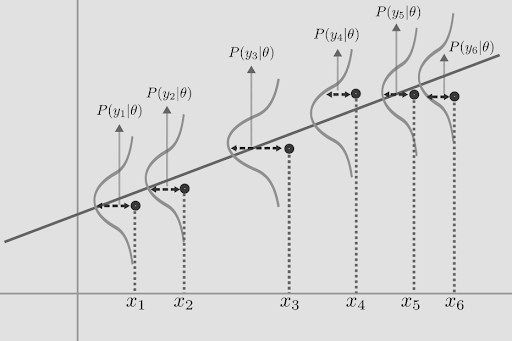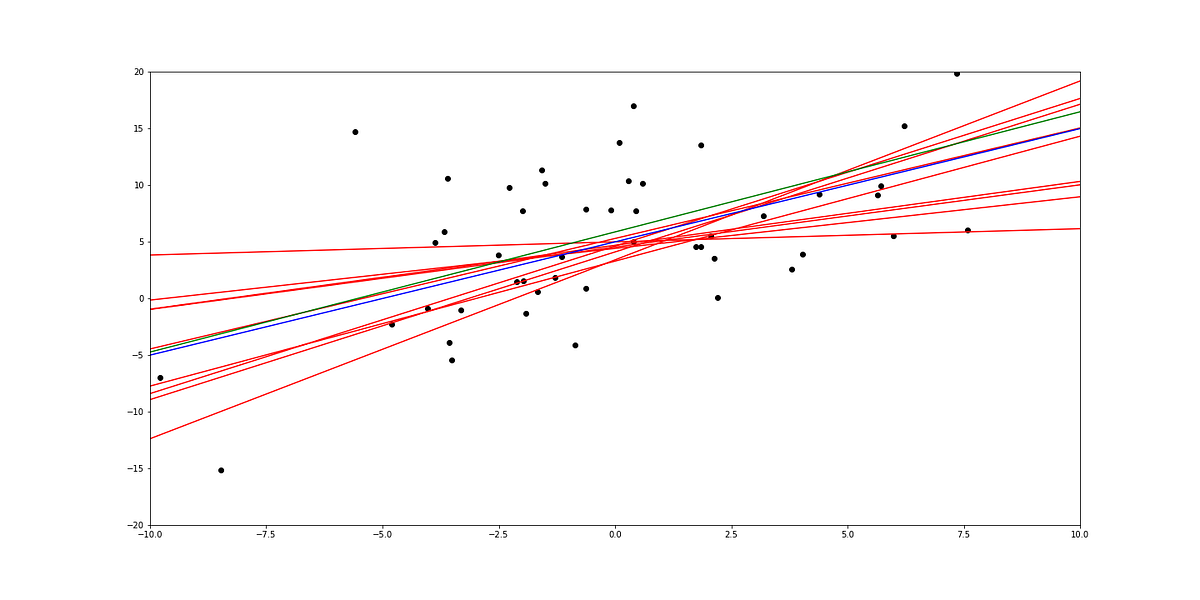1599127200

# Demystifying Model Variance in Linear Regression-1

In this series of blogs I will try to deconstruct ideas in mathematical statistics as I have come to understand them intuitively. This is not a tutorial on Regression analysis but an attempt to bring concepts in Statistics out from the sophistication of mathematical language, within the reach of Data Scientists.

## Setting context

Consider the equation for Linear Regression

y=(β0)+(β1)(x1)+(β2)(x2)+e(~N(0,σ²))

_Here y is regressed variable, __x1 __is the__regressor variable, __β0 __is the intercept, _β1 _is__slope**, e __is the error which is distributed normally with mean _and variance σ². Essentially, it means that y is random variable distributed normally with fixed variance σ² for all values of x1, and its mean is a linear function of x1 → β0+(β1)(x1). See Fig.0**Fig.0, Source

When the model is fitted on any given data using OLS, The coefficients obtained themselves do not give sufficient info. It needs to be evaluated how reliable they are. There can be variables in the model that have high magnitude of the estimated coefficient, yet their “statistical significance” is low.

Q: Are we talking about the accuracy of the fitted model?

A:_ No, a model can have high Squared-Error, or low R-squared implying low accuracy and yet highly “statistically significant” variables._

(We shall discuss more about “statistical significance” of variables, Squared-Error and their relation to under/over fitting in later parts, for now let us try to understand the “Variance” of model estimators.)

Q: Are we talking about Var(X1) or Var(X2)?

A:_ No, we are talking about the variance of the estimated coefficients _β0* and β1* _(we shall be using * notation for predicted values)_

Q: How can the coefficients have variance? Isn’t Linear regression supposed to be deterministic? Whenever I fit OLS model on my data, it gives the same coefficients every time

A:_ Let us start by understanding the difference between population and sample. It is assumed that the sample is drawn out of an infinite population that has a fixed distribution. __The data we have is only a sample drawn from the population. __The larger the size of the sample, the more closely it resembles the population. Thus 1)The estimated parameters for a single sample with large number of data points tend towards the actual population. For any finite sample size, we can only imperfectly estimate the population parameters. But, in case of OLS estimators, _2)The average of estimated parameters over a large number of samples tends towards the actual population parameters.

For Simple Linear Regression, the equation y=β0+(β1)(x)+e(~N(0,σ²))****represents the population from which the sample is assumed to have been drawn. Thus β0, β1, σ² are the parameters of the population.

The coefficients obtained by OLS regression _β0* _and** β1* _are only estimates of the actual population parameters based on the given sample.__For every random sample that is drawn from the population, different values of the parameters are estimated. Thus there is _‘Variance’**_ in the estimated parameters. The estimates that are calculated for any given sample could very well have been obtained even if the actual population parameters were different._

#machine-learning #statistics #statistical-analysis #linear-regression #data-science

## Buddha Community1594271340

## A Deep Dive into Linear Regression

Let’s begin our journey with the truth — machines never learn. What a typical machine learning algorithm does is find a mathematical equation that, when applied to a given set of training data, produces a prediction that is very close to the actual output.

Why is this not learning? Because if you change the training data or environment even slightly, the algorithm will go haywire! Not how learning works in humans. If you learned to play a video game by looking straight at the screen, you would still be a good player if the screen is slightly tilted by someone, which would not be the case in ML algorithms.

However, most of the algorithms are so complex and intimidating that it gives our mere human intelligence the feel of actual learning, effectively hiding the underlying math within. There goes a dictum that if you can implement the algorithm, you know the algorithm. This saying is lost in the dense jungle of libraries and inbuilt modules which programming languages provide, reducing us to regular programmers calling an API and strengthening further this notion of a black box. Our quest will be to unravel the mysteries of this so-called ‘black box’ which magically produces accurate predictions, detects objects, diagnoses diseases and claims to surpass human intelligence one day.

We will start with one of the not-so-complex and easy to visualize algorithm in the ML paradigm — Linear Regression. The article is divided into the following sections:

1. Need for Linear Regression

2. Visualizing Linear Regression

3. Deriving the formula for weight matrix W

4. Using the formula and performing linear regression on a real world data set

Note: Knowledge on Linear Algebra, a little bit of Calculus and Matrices are a prerequisite to understanding this article

Also, a basic understanding of python, NumPy, and Matplotlib are a must.

## 1) Need for Linear regression

Regression means predicting a real valued number from a given set of input variables. Eg. Predicting temperature based on month of the year, humidity, altitude above sea level, etc. Linear Regression would therefore mean predicting a real valued number that follows a linear trend. Linear regression is the first line of attack to discover correlations in our data.

Now, the first thing that comes to our mind when we hear the word linear is, a line.

Yes! In linear regression, we try to fit a line that best generalizes all the data points in the data set. By generalizing, we mean we try to fit a line that passes very close to all the data points.

But how do we ensure that this happens? To understand this, let’s visualize a 1-D Linear Regression. This is also called as Simple Linear Regression

#calculus #machine-learning #linear-regression-math #linear-regression #linear-regression-python #python1592023980

## 5 Regression algorithms: Explanation & Implementation in Python

Take your current understanding and skills on machine learning algorithms to the next level with this article. What is regression analysis in simple words? How is it applied in practice for real-world problems? And what is the possible snippet of codes in Python you can use for implementation regression algorithms for various objectives? Let’s forget about boring learning stuff and talk about science and the way it works.

#linear-regression-python #linear-regression #multivariate-regression #regression #python-programming1598352300

## Regression: Linear Regression

Machine learning algorithms are not your regular algorithms that we may be used to because they are often described by a combination of some complex statistics and mathematics. Since it is very important to understand the background of any algorithm you want to implement, this could pose a challenge to people with a non-mathematical background as the maths can sap your motivation by slowing you down.In this article, we would be discussing linear and logistic regression and some regression techniques assuming we all have heard or even learnt about the Linear model in Mathematics class at high school. Hopefully, at the end of the article, the concept would be clearer.

**Regression Analysis **is a statistical process for estimating the relationships between the dependent variables (say Y) and one or more independent variables or predictors (X). It explains the changes in the dependent variables with respect to changes in select predictors. Some major uses for regression analysis are in determining the strength of predictors, forecasting an effect, and trend forecasting. It finds the significant relationship between variables and the impact of predictors on dependent variables. In regression, we fit a curve/line (regression/best fit line) to the data points, such that the differences between the distances of data points from the curve/line are minimized.

#regression #machine-learning #beginner #logistic-regression #linear-regression #deep learning1601431200

## Polynomial Regression — The “curves” of a linear model

The most glamorous part of a data analytics project/report is, as many would agree, the one where the Machine Learning algorithms do their magic using the data. However, one of the most overlooked part of the process is the preprocessing of data.

A lot more significant effort is put into preparing the data to fit a model on rather than tuning the model to fit the data better. One such preprocessing technique that we intend to disentangle is Polynomial Regression.

#data-science #machine-learning #polynomial-regression #regression #linear-regression1599127200

## Demystifying Model Variance in Linear Regression-1

In this series of blogs I will try to deconstruct ideas in mathematical statistics as I have come to understand them intuitively. This is not a tutorial on Regression analysis but an attempt to bring concepts in Statistics out from the sophistication of mathematical language, within the reach of Data Scientists.

## Setting context

Consider the equation for Linear Regression

y=(β0)+(β1)(x1)+(β2)(x2)+e(~N(0,σ²))

_Here y is regressed variable, __x1 __is the__regressor variable, __β0 __is the intercept, _β1 _is__slope**, e __is the error which is distributed normally with mean _and variance σ². Essentially, it means that y is random variable distributed normally with fixed variance σ² for all values of x1, and its mean is a linear function of x1 → β0+(β1)(x1). See Fig.0**Fig.0, Source

When the model is fitted on any given data using OLS, The coefficients obtained themselves do not give sufficient info. It needs to be evaluated how reliable they are. There can be variables in the model that have high magnitude of the estimated coefficient, yet their “statistical significance” is low.

Q: Are we talking about the accuracy of the fitted model?

A:_ No, a model can have high Squared-Error, or low R-squared implying low accuracy and yet highly “statistically significant” variables._

(We shall discuss more about “statistical significance” of variables, Squared-Error and their relation to under/over fitting in later parts, for now let us try to understand the “Variance” of model estimators.)

Q: Are we talking about Var(X1) or Var(X2)?

A:_ No, we are talking about the variance of the estimated coefficients _β0* and β1* _(we shall be using * notation for predicted values)_

Q: How can the coefficients have variance? Isn’t Linear regression supposed to be deterministic? Whenever I fit OLS model on my data, it gives the same coefficients every time

A:_ Let us start by understanding the difference between population and sample. It is assumed that the sample is drawn out of an infinite population that has a fixed distribution. __The data we have is only a sample drawn from the population. __The larger the size of the sample, the more closely it resembles the population. Thus 1)The estimated parameters for a single sample with large number of data points tend towards the actual population. For any finite sample size, we can only imperfectly estimate the population parameters. But, in case of OLS estimators, _2)The average of estimated parameters over a large number of samples tends towards the actual population parameters.

For Simple Linear Regression, the equation y=β0+(β1)(x)+e(~N(0,σ²))****represents the population from which the sample is assumed to have been drawn. Thus β0, β1, σ² are the parameters of the population.

The coefficients obtained by OLS regression _β0* _and** β1* _are only estimates of the actual population parameters based on the given sample.__For every random sample that is drawn from the population, different values of the parameters are estimated. Thus there is _‘Variance’**_ in the estimated parameters. The estimates that are calculated for any given sample could very well have been obtained even if the actual population parameters were different._

#machine-learning #statistics #statistical-analysis #linear-regression #data-science